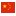# Representation of results of particle size analysis– Part 2: Calculation of average particle sizes/diameters and moments from particle size distributions

Standard Number GB/T 15445.2-2006 Standardization Administration of ChinaNational Guide | Practice FEB 2006 Published
ABSTRACT
his standard applies to provide some formulas to from a given particle size distribution to calculate the average or mean diameter of the value of the second moment. In this standard assumes that the particle size distribution histogram representation. If the particle size distribution in the form of analytic functions, the corresponding mathematical treatment applies. In this standard, it is also assumed that the particles of any shape can be its equivalent spherical diameter x, as measured with the same volume of particles to represent the diameter of the ball.